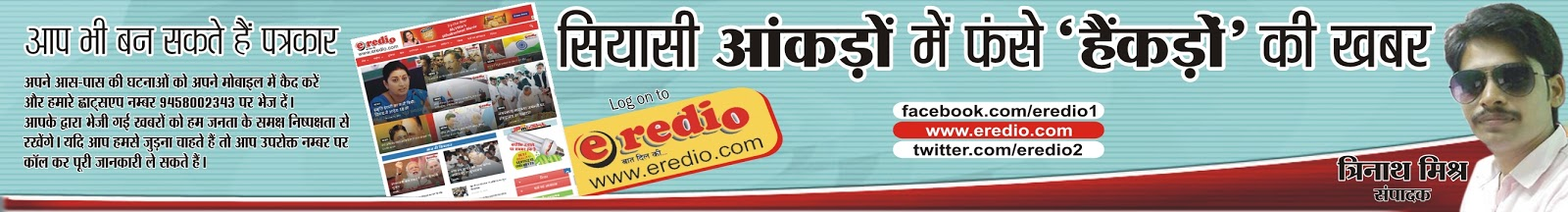# NANHI KALAM नन्ही कलम

'No candle looses its light while lighting up another candle'So Never stop to helping Peoples in your life.## Dec 24, 2009

If

A B C D E F G H I J K L M N O P Q R S T U V W X Y Z
is equal to
1 2 3 4 5 6 7 8 9 10 11 12 13 14 15 16 17 18 19 20 21 22 23 24 25 26

Then,

Hard WorkH+A+R+D+W+O+R+K
8+1+18+4+23+15+18+11 = 98%

Knowledge
K+N+O+W+L+E+D+G+E
11+14+15+23+12+5+4+7+5 = 96%

Love
L+O+V+E
12+15+22+5 = 54%

Luck
L+U+C+K
12+21+3+11 = 47%

most of us think this is the most important ??? )

Then what makes 100% ?

Is it Money ? .. NO ! ! !

M+O+N+E+Y
13+15+14+5+25 = 72%

Leadership ? .... NO ! ! !

L+E+A+D+E+R+S+H+I+P
12+5+1+4+5+18+19+9+16 = 89%

Every problem has a solution, only if we perhaps change our attitude.

To go to the top,

what we really need to go further... a bit more...

ATTITUDE
A+T+T+I+T+U+D+E
1+20+20+9+20+21+4+5 = 100%